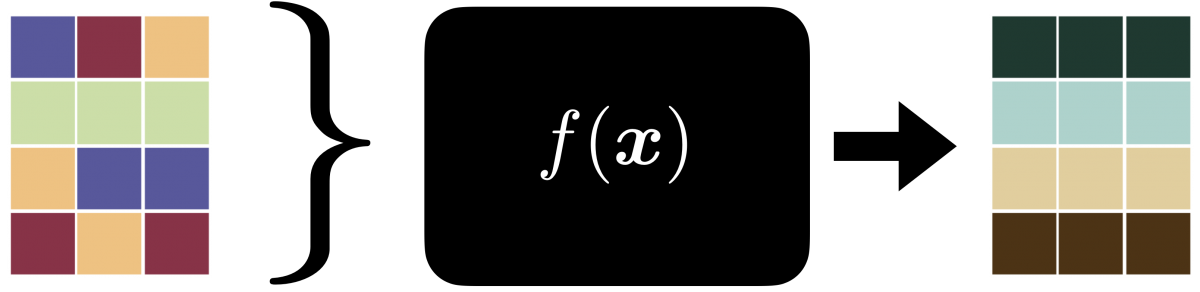We use standard linear models to solve many imaging problems. Especially, when we know the exact geometry of the measurement process, this is true in imaging large objects where we can control the object's orientations and position. While this has helped in several breakthroughs, we look at extending the methodology further, especially when the dynamics of the objects that are being measured are unknown. This is true while imaging molecules or smaller entities. We cannot precisely measure the orientation of the objects or keep the objects fixed. In general, we can model the unknowns as:

$$y = TAx$$

We look at the permutation invariances, which come in the context of sets. $$y = \Pi Ax$$

Apart from training neural nets with all the data variants, we look at efficient network architectures to make them invariant or equivariant to such model changes.## Publications

### 2019

Permutations unlabeled beyond sampling unknown
IEEE Signal Processing Letters, 2019
@article{dokmanic2019permutations,
title={Permutations unlabeled beyond sampling unknown},
author={Dokmani{\'c}, Ivan},
journal={IEEE Signal Processing Letters},
volume={26},
number={6},
pages={823--827},
year={2019},
publisher={IEEE}
}